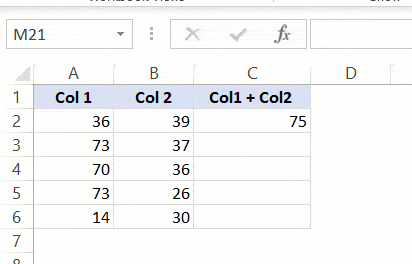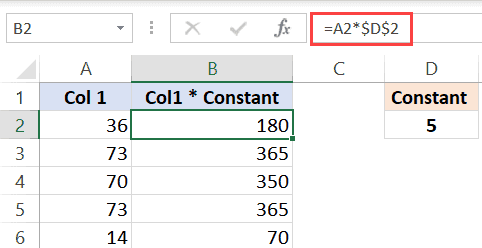# What does \$ (dollar sign) mean in Excel Formulas?

If you have worked with Excel formulas, I am sure you have noticed that sometimes there is a \$ symbol as a part of the cell references.

## What does \$ mean in Excel formulas?

One of the things that make Excel such a powerful tool is the ability to refer to cells/ranges and use these in formulas.

And when you copy these formulas, these cell references can adjust automatically (or should I say automatically).

Below is an example where I copy the cell C2 (which has a formula) and paste it in C3.You can see that the formula adjusts the references when I copy and paste it. While in the formula in cell C2 refers to A2 and B2, the one in C3 refers to A3 and B3.

This is called relative reference where the references adjust based on the cell in which it has been applied.

But what if you don’t want some cells to adjust the reference?

What if you want to copy the formula, but don’t want the cell reference to change?

…. introducing the \$ sign.

When you use a \$ sign before the cell reference (such as \$C\$2), you’re telling Excel to keep referring to cell C3 even when you copy and paste the formula.

Now you can use the dollar (\$) sign in three different ways, which means that there are three types of references on Excel.

## Shortcut to add \$ Sign to Cell References

There are two ways you can add the \$ sign to a cell reference in Excel.

You can either do it manually (i.e., go into the edit mode in a cell by double-clicking on it or using F2, placing the cursor where you want the \$ sign and then typing it manually).

Or you can use the keyboard shortcut

` F4 `

To use this shortcut, simply place the cursor on the cell reference where you want to add the dollar sign and press is once. You will notice that it will change the reference by adding/removing the \$ sign (based on what’s the original reference).

For example, suppose you have the reference C2 in a cell. Here is how the F4 shortcut would work:

• Press F4 one time – C2 will change to \$C\$2
• Press F4 two times – C2 will change to C\$2
• Press F4 three times – C2 will change to \$C2
• Press F4 four times – C2 will change back to C2

## Types of References in Excel

There are three types of references in Excel:

• Relative references
• Absolute references
• Mixed references

In relative references, you don’t use a dollar (\$) sign in the references at all.

In mixed references, you use the dollar sign (\$) only once (such as \$C3 or C\$3)

In absolute reference, you use the dollar sign in twice in a reference (such as \$C\$3).

Let me quickly explain each of these with a simple example.

### Relative reference

Relative reference is where you don’t use a dollar (\$) sign at all.

And when you copy a cell that has a relative reference, it will change and adjust based on the cell where you copy it.

Below is the same example again, where the references adjust as soon as we copy and paste the cell that has the formula.### Absolute reference

In absolute references, you have the \$ sign before the row number and the column alphabet (example \$C\$3)

When you use this in formulas, it will not change the reference

when you copy and paste the cell. This could be useful when you have some value that needs to remain constant (such as time period or interest rates, etc.)

Below is an example where I have a value in cell D2 which needs to remain constant (and not change when we copy-paste the formulas).By using \$D\$2, we make sure that it doesn’t change when we copy-paste the cell with the formula.

Note that this example has both, relative cell reference (without the \$ sign) and absolute cell reference (with two \$ signs).

Note: If you’re wondering why not simply hard code the value instead of using the absolute cell reference (the one with two dollar signs). While you can use the value itself, in the future, if you have to change the value in formulas, you will have to manually do it. Instead, you can simply change the value in C3, and all the formulas would automatically update.

### Mixed reference

These are a little more complicated than the rest two.

In mixed cell references, you will have only one dollar sign (for example – \$C3 or C\$3)

When you add a dollar sign in front of the column alphabet (C in this example), it locks the column only. This means that if you copy-paste the formula that uses \$C3, the column would not change, but the row can change.

And when you add a dollar sign in front of the row number (3 in this example), it locks the column only. This means that if you copy-paste the formula that uses C\$3, the row would not change, but the column can change.

Here is a good article that goes in-depth about the mixed cell references in Excel.

## Summary

A dollar sign means that the part of the cell reference before which it has been used is anchored or fixed.

Below is a quick summary of what \$ means in Excel formulas:

1. \$A\$1 – always refers to column A and row 1
2. \$A1 – Column A is fixed and will not change, but the row is allowed to change as the formula is copied
3. A\$1 – Row 1 is fixed and will not change, but the column is allowed to change as the formula is copied.
4. \$A\$1:\$A\$100 – always refers to the range A1:A100

I hope this article helps you understand what the \$ sign means in Excel and how to use it.

Other Excel tutorials you may find useful: